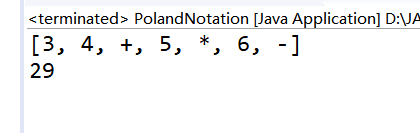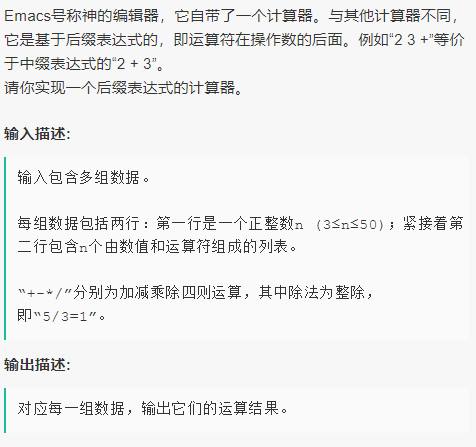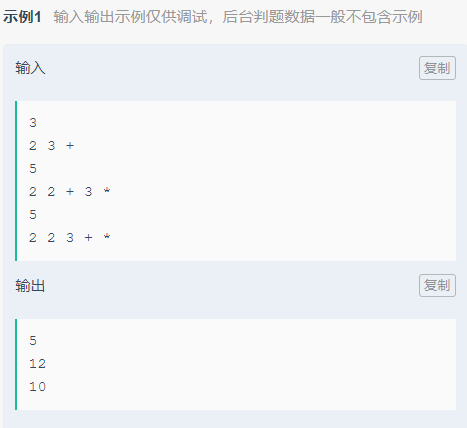• java使用后缀表达式实现计算器，其中有将一般数学运算式（7-9+5/5-5*6）转换成后缀表达式的方法，以及后缀表达式的求解方法
• 输入一个逆波兰表达式(后缀表达式), 使用栈(Stack), 计算其结果 支持小括号和多位数整数, 因为这里我们主要针对于数据结构, 因此计算器进行简化, 只支持对整数的计算 思路分析 代码完成 package com.beyond.stack; ...
逆波兰计算器的实现
输入一个逆波兰表达式(后缀表达式), 使用栈(Stack), 计算其结果支持小括号和多位数整数, 因为这里我们主要针对于数据结构, 因此计算器进行简化, 只支持对整数的计算思路分析代码完成
package com.beyond.stack;

import java.util.ArrayList;
import java.util.List;
import java.util.Stack;

public class PolandNotation {

public static void main(String[] args) {
// 先定义一个逆波兰表达式
// (3+4)*5-6  => 3 4 + 5 * 6 -
// 为了方便, 逆波兰表达式 的数字和符号使用空格隔开
String suffixExpression = "3 4 + 5 * 6 -";

// 思路:
// 1. 先将 "3 4 + 5 * 6 -" => 放到 ArrayList中
// 2. 将 ArrayList 传递给一个方法, 遍历ArrayList 配合 栈 完成计算

List<String> rpnList = getListString(suffixExpression);
System.out.println(rpnList);
System.out.println(calculate(rpnList));
}

// 将一个逆波兰表达式, 依次将数据和运算符 放入 ArrayList 中
public static List<String> getListString(String suffixExpression){
// 将suffixExpression 分割
String split [] = suffixExpression.split(" ");
List<String> list = new ArrayList<String>();
for(String ele: split) {
}
return list;
}

// 完成对逆波兰表达式的运算
/**
* 1. 从左到右扫描, 将3和4压入堆栈;
* 2. 遇到 ＋ 运算符, 因此弹出 4和3 (4 为栈顶元素,3为次栈顶元素), 计算 3+4 的值, 得7, 再将7 入栈
* 3. 将 5 入栈
* 4. 接下来是 * 运算符, 因此 弹出 5和7, 计算出 7*5 = 35, 将35 入栈
* 5. 将6入栈
* 6. 最后是 - 运算符, 计算出 35 -6 的值, 即29, 由此得出最终结果
*/
public static int calculate(List<String> ls) {
// 创建个栈, 一个栈即可
Stack<String> stack = new Stack<String>();
// 遍历 ls
for(String item: ls) {
// 使用一个正则表达式来取出数
if (item.matches("\\d+")) {  // 匹配的是多位数
// 入栈
stack.push(item);
}else {
// pop 出两个数, 并运算, 再入栈
int num2 = Integer.parseInt(stack.pop());
int num1 = Integer.parseInt(stack.pop());
int res = 0;
if (item.equals("+")) {
res = num1 + num2;
}else if (item.equals("-")) {
res = num1 - num2;
}else if (item.equals("*")) {
res = num1 * num2;
}else if (item.equals("/")) {
res = num1 / num2;
}else {
throw new RuntimeException("运算符有误!");
}

// 把 res 入栈
stack.push(String.valueOf(res)); // 转成字符串, 还可以 Integer.toString(res) 或者使用 +""

}
}

// 最后留在 stack 中的数据就是运算结果
return Integer.parseInt(stack.pop());

}

}展开全文数据结构
• 本文用Java实现了基于后缀表达式的简易计算器
直接上代码
package cn.john.cal;

/**
*
* @ClassName: Operators
*
* @Description: 操作符枚举
*
* @author: John
*
* @date: 2017年5月9日 下午22:05:16
*
*
*/
public enum OperatorsEnum {

PLUS(0, '+'), MINUS(0, '-'), MULTIPLY(1, '*'), DIVIDE(1, '/'), MODULAR(1, '%'), LEFT_BRACKET(2,
'('), RIGHT_BRACKET(2, ')');

public Integer prior;// 优先级
public Character operator;// 操作符

private OperatorsEnum(int prior, char operator) {
this.prior = prior;
this.operator = operator;
}

public String toString() {
return String.valueOf(operator);
}

}
package cn.john.cal;

import java.util.Scanner;

/**
*
* @ClassName: Cal
*
* @Description:
*               <p>
*               基于后缀表达式的简易计算器，目前支持个位数加、减、乘、除、模和括号六种运算。
*               <p>
*               中缀表达式->后缀表达式 操作符入栈
*               <p>
*               后缀表达式->计算值 操作数入栈
*
* @author: John
*
* @date: 2017年5月9日 下午7:55:58
*
*/
public class Cal {

/**

* @Title: toPostFix

* @Description: 将中缀表达式转换为后缀表达式

* @param infix
* @return

* @return: String

*/
public String toPostFix(String infix) {
// 算式字符数组
char[] ch = infix.trim().toCharArray();
StringBuilder sb = new StringBuilder();

OperatorsEnum op = null;

for (int i = 0; i < ch.length; i++) {
// 对每个算式字符，检查它是不是操作符
if ((op = isOperator(ch[i])) == null) {
sb.append(ch[i]);
} else {
// 右括号
// 持续弹出栈顶元素直到遇到左括号，但是不输出左括号
if (op.equals(OperatorsEnum.RIGHT_BRACKET)) {
// 如果不是左括号，持续弹出并输出
while (!stack.peek().equals(OperatorsEnum.LEFT_BRACKET)) {
sb.append(stack.pop());
}
// 此时栈顶元素为左括号，直接弹出，不输出
stack.pop();
} else {
// 非右括号
// 1、弹出并输出所有高优先级或者同等优先级，直到遇到低优先级或者左括号为止
// 上面的弹出语句有可能将栈弹空，检查stack的size避免NPE
while (stack.size() > 0 && stack.peek().prior >= op.prior
&& !stack.peek().equals(OperatorsEnum.LEFT_BRACKET)) {
sb.append(stack.pop());
}
// 2、将当前操作符入栈
stack.push(op);
}
}
}
// 弹出所有栈中剩余操作符
while (stack.size() > 0) {
sb.append(stack.pop());
}

return sb.toString();
}

/**

* @Title: calc

* @Description: 计算后缀表达式的值

* @param postfix
* @return

* @return: double

*/
public double calc(String postfix) {
char[] ch = postfix.toCharArray();
OperatorsEnum op = null;
for (int i = 0; i < ch.length; i++) {
if ((op = isOperator(ch[i])) == null) {
// 不是操作符，将当前数值入栈
stack.push(Double.parseDouble(String.valueOf(ch[i])));
} else {
// 是操作符，进行计算
double b = stack.pop();
double a = stack.pop();
switch (op) {
case PLUS:
stack.push(a + b);
break;
case MINUS:
stack.push(a - b);
break;
case MULTIPLY:
stack.push(a * b);
break;
case DIVIDE:
stack.push(a / b);
break;
case MODULAR:
stack.push(a % b);
break;
default:
break;
}
}
}
return stack.pop();
}

/**

* @Title: isOperator

* @Description: 判断字符是否为操作符

* @param ch
* @return

* @return: OperatorsEnum

*/
private OperatorsEnum isOperator(char ch) {
for (OperatorsEnum op : OperatorsEnum.values()) {
if (ch == op.operator) {
return op;
}
}
return null;
}

// test

/**

* @Description: 终端输入算式

* @return

* @return: String

*/
Scanner sc = new Scanner(System.in);
String equation = sc.nextLine();
sc.close();
return equation;
}

public static void main(String[] args) {
Cal c = new Cal();
System.out.println("Please input an equation,press ENTER to submit!");
String s = c.toPostFix(infix);
System.out.println("postfix: " + s);
System.out.println("Result: "+infix + "=" + c.calc(s));
}
}
展开全文java
• 对于较为复杂的四则运算表达式，例如：9-(5-2.4)*2+6，计算这样的表达式必须考虑计算的优先级，计算机通常很难直接计算这样的表达式，而为了实现计算这种表达式的功能，可以先将其转化为后缀表达式，即所有的运算符...
对于较为复杂的四则运算表达式，例如：9-(5-2.4)*2+6，计算这样的表达式必须考虑计算的优先级，计算机通常很难直接计算这样的表达式，而为了实现计算这种表达式的功能，可以先将其转化为后缀表达式，即所有的运算符都是在运算数的后面，即先把该表达式转换为“9 5 2.4 - 2 * - 6 +”再进行计算，我们先从简单的开始理解。
后缀表达式的计算
对于后缀表达式，用计算机计算起来就容易多了，从左向右遍历，遇到数字就进栈，遇到符号就将栈顶的两个元素出栈，并进行运算，运算得到的结果再进栈，对于“9 5 2.4 - 2 * - 6 +”具体运算过程如下：实现代码：
def Calculate(suffix_count):
count_list = []
for co in suffix_count:
if symbol_list.count(co) == 0:  # 判断是否为数字
count_list.append(float(co))
else:
a = count_list.pop()
b = count_list.pop()
if co == '*':
count_list.append(b * a)
elif co == '/':
count_list.append(b / a)
elif co == '+':
count_list.append(b + a)
elif co == '-':
count_list.append(b - a)
return count_list

中缀表达式转后缀表达式
计算后缀表达式的规则很容易，相信大家都能看懂，而该程序的核心难点是中缀表达式转后缀表达式。我们平时常用的四则运算式的表示法即为中缀表达式，中缀表达式转后缀表达式规则：从左到右遍历中缀表达式，若是数字则输出到后缀表达式中，若是运算符，则判断其与栈顶符号的优先级，是右括号或优先级低于栈顶符号则栈顶元素依次出栈并输出到后缀表达式中，并将当前符号进栈，若为左括号或优先级高于栈顶元素则直接进栈，一直到最终输出后缀表达式为止。
实现代码
symbol_list = ['(', ')', '+', '-', '*', '/']
def MiddleToBack(middle_equation):#中缀转后缀
symbol_flag=0#运算符优先级标志位
count_flag=0#数字标志位
suffix_count=[]
suffix_symbol=[]
otherai=''
for ch in middle_equation:
if '0'<=ch<='9' or ch=='.':
if count_flag==0:#判断若上一位不是数字
suffix_count.append(ch)#在列表加上新的数字
else:
suffix_count[-1]=suffix_count[-1]+ch#在列表末尾的元素加上一位
count_flag=1
elif symbol_list.count(ch):
count_flag=0
if ch=='(':
symbol_flag=0
suffix_symbol.append(ch)
elif ch==')':
out=''#初始化out
while out!='(':
out=suffix_symbol.pop()
if out!='(':
suffix_count.append(out)
symbol_flag = 0
elif ch == '*':
MultiplyAndDivide(symbol_flag,suffix_symbol,suffix_count,ch)
symbol_flag=1
elif ch == '/':
MultiplyAndDivide(symbol_flag,suffix_symbol,suffix_count,ch)
symbol_flag=1
elif ch=='+':
symbol_flag=0
elif ch=='-':
symbol_flag=0
else:
print('error')
break
while len(suffix_symbol):#把符号栈中剩下的依次给予算式栈
suffix_count.append(suffix_symbol.pop())
return suffix_count
def MultiplyAndDivide(symbol_flag,suffix_symbol,suffix_count,ch):#* /
if symbol_flag:
suffix_count.append(suffix_symbol.pop())
suffix_symbol.append(ch)
if suffix_symbol.count('('):
otherai = suffix_symbol[-1]
while otherai != '(':
suffix_count.append(otherai)
suffix_symbol.pop()
otherai = suffix_symbol[-1]
else:
if len(suffix_symbol):
symbol_last = suffix_symbol[-1]
while symbol_last == '*' or symbol_last == '/':
suffix_count.append(suffix_symbol.pop())
if len(suffix_symbol):
symbol_last = suffix_symbol[-1]
else:
symbol_last = ''
while len(suffix_symbol):
suffix_count.append(suffix_symbol.pop())
suffix_symbol.append(ch)

算法思路参考《大话数据结构》，感觉代码写的挺乱的，也有点繁琐，但居然也通过了牛客网的测试，感兴趣的小伙伴也去练习一下试试吧。https://www.nowcoder.com/profile/706249245/myFollowings/detail/16458229
展开全文算法 python 数据结构
• 循环输入，获取逆波兰表达式（后缀表达式），然后进行以下步骤，直到测试完所有的测试用例： 遇到数字字符串，将该数字字符串转化为数字然后入栈。利用int atio(const char ptr）将数字类型的字符串转换成整形数据...解题思路
循环输入，获取逆波兰表达式（后缀表达式），然后进行以下步骤，直到测试完所有的测试用例：
遇到数字字符串，将该数字字符串转化为数字然后入栈。利用int atio(const char ptr）将数字类型的字符串转换成整形数据；它包含在stdlib.h头文件中； 这里的参数类型是char所以这里要将string转化为c类型的string，在进行转化。遇到操作符时，从栈顶取两个数字，然后进行该运算符所对应运算，完成好将结果入栈。
NOTE：根据逆波兰表达式定义：先取到的数字为运算符的右操作数。 继续1和2，直到处理完所有的字符串，最终栈顶元素即为所要结果。
代码
#include<iostream>
#include<stack>
#include<string>
#include<cstdlib>
using namespace std;

int main()
{
int n;
while(cin>>n)
{
if(n<3 || n>50)
return 0;

stack<int> st;
int num1=0;
int num2=0;
string str;

for(int i=0;i<n;i++)
{
cin>>str;
if((str>='0') && (str<='9'))
st.push(atoi(str.c_str()));
else
{
num1=st.top();
st.pop();
num2=st.top();
st.pop();
if(str=="+")
{
num1=num2+num1;
st.push(num1);
}
else if(str=="-")
{
num1=num2-num1;
st.push(num1);
}
else if(str=="*")
{
num1=num2*num1;
st.push(num1);
}
else if(str=="/")
{
num1=num2/num1;
st.push(num1);
}

}
}
cout<<st.top()<<endl;
}

return 0;
}

展开全文• 后缀表达式对于人来说是比较难理解的，但是对于计算机来说，缺失比较简单易懂的结构，这主要是由于计算机内存是栈式结构。当我们知道一个表达式的后缀表达式的时候，... * 逆波兰表达式计算器 */ public class PolandN逆波兰式
• * 中缀表达式转后缀表达式，需要借助栈来存放操作符 * &lt;p&gt; * 1. 遇到的是数字，直接输出 * 2. 遇到的第一个操作符进栈 * 3. 遇到"("进栈 * 4. 遇到其他操作符oper...
• 中缀表达式改后缀表达式，而且有计算器功能，一般的加减乘除都能
• 逆波兰表达式又叫做后缀表达式。逆波兰表示法是波兰逻辑学家J・卢卡西维兹(J・ Lukasiewicz)于1929年首先提出的一种表达式的表示方法  。后来,人们就把用这种表示法写出的表达式称作“逆波兰表达式”。逆波兰...列表 stack 算法
• 后缀表达式是相较于中缀表达式而言的，像我们平时写的2+3*(4-(5+6))/7就是一个中缀表达式，那么如何将之变为后缀表达式呢？后缀表达式如何用来求解呢？ 先来第一个问题（中缀->后缀）： 变为后缀表达式方法...
• 它遵循”先进后出，后进先出“原则，底层一般由数组或者单向链表实现的，接下来介绍一下栈应该场景，用栈实现中缀表达式转后缀表达式（逆波兰表达式）和实现简单计算器计算。 2.中缀表达式转后缀表达式：中缀表达式...数据结构
• 后缀表达式实现简单计算器（逆波兰计算器 ） 中缀表达式：(3+4)×5-6 ------> 后缀表达式： 3 4 + 5 × 6 - 输入一个逆波兰表达式(后缀表达式)，使用栈(Stack), 计算其结果 思路分析： 举个栗子： (3+4)×5-6 ...数据结构 stack
• // 完成将一个中缀表达式转换成后缀表达式的功能 // 说明 // 1. 1+((2+3)*4)-5 ==> 转成 1 2 3 + 4 * + 5 - // 2. 因为直接对字符串进行操作不方便，因此先将"1+((2+3)*4)-5" => 中缀表达式对应的list // ...
• 后缀表达式在计算机中运算是按照以下规则： 1 先从左到右扫描表达式，遇到数字时，则将该数字压进栈中。 2 遇到运算符时，则把栈顶的两个数（栈顶元素和次顶元素）弹出，与运算符进行相应的计算，并把计算结果...数据结构 java stack
• 完成对逆波兰表达式的运算 1)从左至右扫描，将3和4压入堆栈; 2)遇到+运算符，因此弹出4和3 (4为栈项元素，3为次项元素)，计算出3+4的值，得7,再将7入楼; ...后缀表达式适合计算式进行运算，但是...
• //中缀表达式转后缀表达式的函数 void postfix ( char * , char * ) ; double read_number ( char * dest , int * i ) ; double answer ( char * dest ) ; void match ( char * ...
• 源代码 博文链接：https://sylinx.iteye.com/blog/215322
• 模拟栈 stack.h#inndef _STACK_H #define _STACK_H typedef char Bool; typedef int StackType; typedef struct stack stack; #define false 0 #define true 1 #define MAXSIZE 100 ... StackType buf
• 计算器代码： 管理员输入常见算术表达式，先转换成逆波兰，计算机再计算，上一篇中介绍过顺序栈的初始化，直接贴main里的处理函数 /* 逆波兰表示 是符号则运算 是数字则入栈 输入以#结束 */ /* 输入中缀...
• 中缀转后缀表达式思路 新建一个栈辅助转换表达式 遍历中缀表达式： 如果是数字直接加入表达式尾端 遇到括号时： （1）左括号：将左括号压入栈中，继续遍历 （2）右括号：将栈中遇到左括号之前的符号依次添加到......